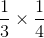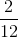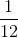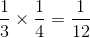# ISEE Lower Level Quantitative : Operations with fractions and whole numbers

## Example Questions

← Previous 1 3 4 5 6 7 8 9 18 19

### Example Question #2 : Multiply A Fraction Or Whole Number By A Fraction: Ccss.Math.Content.5.Nf.B.4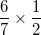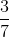Explanation:

When we multiply fractions, we multiply the numerator by the numerator and the denominator by the denominator.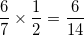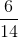can be reduced by dividing both sides by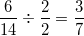### Example Question #3 : Multiply A Fraction Or Whole Number By A Fraction: Ccss.Math.Content.5.Nf.B.4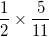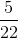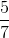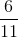Explanation:

When we multiply fractions, we multiply the numerator by the numerator and the denominator by the denominator.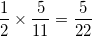### Example Question #1681 : Ssat Middle Level Quantitative (Math)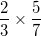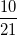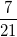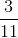Explanation:

When we multiply fractions, we multiply the numerator by the numerator and the denominator by the denominator.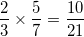### Example Question #5 : Multiply A Fraction Or Whole Number By A Fraction: Ccss.Math.Content.5.Nf.B.4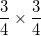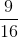Explanation:

When we multiply fractions, we multiply the numerator by the numerator and the denominator by the denominator.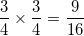### Example Question #6 : Multiply A Fraction Or Whole Number By A Fraction: Ccss.Math.Content.5.Nf.B.4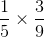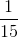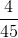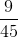Explanation:

When we multiply fractions, we multiply the numerator by the numerator and the denominator by the denominator.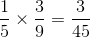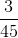can be reduced by dividing both sides by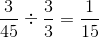### Example Question #7 : Multiply A Fraction Or Whole Number By A Fraction: Ccss.Math.Content.5.Nf.B.4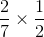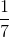Explanation:

When we multiply fractions, we multiply the numerator by the numerator and the denominator by the denominator.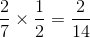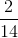can be reduced by dividing both sides by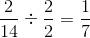### Example Question #8 : Multiply A Fraction Or Whole Number By A Fraction: Ccss.Math.Content.5.Nf.B.4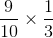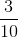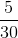Explanation:

When we multiply fractions, we multiply the numerator by the numerator and the denominator by the denominator.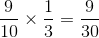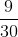can be reduced by dividing both sides by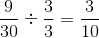### Example Question #9 : Multiply A Fraction Or Whole Number By A Fraction: Ccss.Math.Content.5.Nf.B.4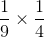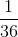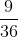Explanation:

When we multiply fractions, we multiply the numerator by the numerator and the denominator by the denominator.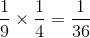### Example Question #10 : Multiply A Fraction Or Whole Number By A Fraction: Ccss.Math.Content.5.Nf.B.4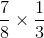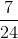Explanation:

When we multiply fractions, we multiply the numerator by the numerator and the denominator by the denominator.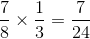### Example Question #1 : Operations With Fractions And Whole Numbers